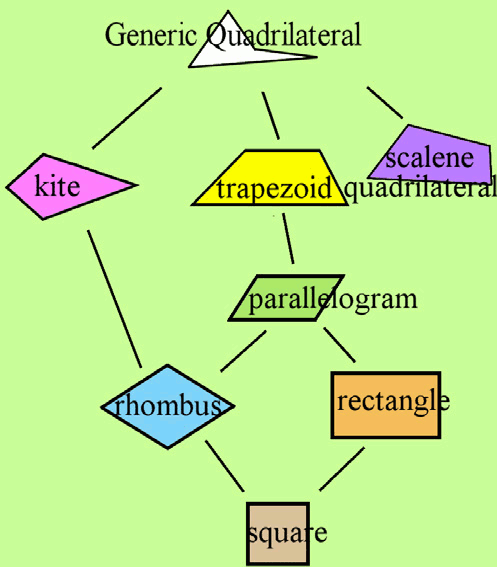^
You are here: HomeLessons → Trapezoid definition

# What is the definition of a trapezoid? One set of parallel lines OR at least one pair of parallel lines?

Several people have questioned me about the definition of a trapezoid that appears in Math Mammoth grade 5 curriculum:

A trapezoid has at least one pair of parallel sides. It may have two!

You can easily find other definitions for a trapezoid which indicate it only has ONE pair of parallel sides. So, people get confused.

This is one of those rare instances where there exist two types of definitions for a shape. The one I used is an "inclusive" definition, because according to it, squares and rectangles and parallelograms are INCLUDED... they are trapezoids also.

But some of my customers have learned the "exclusive" definition, which says a trapezoid has exactly one pair of parallel lines (not two). Using this definition, squares, parallelograms, and rectanges won't qualify.

Mathematicians prefer the inclusive definition (the one that says "at least one pair of parallel lines"). Why? Because it makes their theorems shorter to state. If something is true of all trapezoids, that one word "trapezoid" captures all the shapes with at least one pair of parallel lines, and they don't have to state that it is true for "trapezoids, parallelograms, rhombi, rectangles, and squares". It just makes things simpler.

The same is true in the case of a square versus rectangle. Little children often think in terms of the EXCLUSIVE definitions, and view squares as not rectangles. To them, a rectangle cannot have all equal sides. But, as you probably know, the inclusive definition (a rectangle is a shape with four sides and four right angles) is the prevailing one in the adult world, and squares are classified as rectangles.

The reason I used the inclusive definition in Math Mammoth wasn't so much because of theorems related to trapezoids (we don't really study any in elementary math). It was because that definition allows us to draw this neat quadrilateral classification, or tree diagram:Notice, the trapezoid is presented as ABOVE, or as a "parent" of a parallelogram. In other words, a parallelogram is a "child" of a trapezoid. It means all parallelograms ARE trapezoids... they belong to the "trapezoid" family. Similarly, all rhombi are parallelograms. And all squares are reactangles, are parallelograms, are trapezoids.

Inclusive Definitions: Trapezoids from Dr. Math

Definition of a trapezoid from Wolfram Mathworld
Wolfram Mathworld is highly regarded by mathematicians, and their page presents the typical definition preferred by them: "A trapezoid is a quadrilateral with two sides parallel." (This is a typical way to word a definition in mathematics, and it means a trapezoid has at least two sides that are parallel, not that it has exactly two sides that hare parallel.)

Inclusive vs exclusive definition of a trapezoid at Wikipedia

By Maria Miller

WAIT!

Receive my monthly collection of math tips & resources directly in your inbox — and get a FREE Math Mammoth book!You can unsubscribe at any time.

### Math Mammoth Tour

Confused about the different options? Take a virtual email tour around Math Mammoth! You'll receive:

An initial email to download your GIFT of over 400 free worksheets and sample pages from my books. Six other "TOURSTOP" emails that explain the important things and commonly asked questions concerning Math Mammoth curriculum. (Find out the differences between all these different-colored series!)

This way, you'll have time to digest the information over one or two weeks, plus an opportunity to ask me personally about the curriculum.
A monthly collection of math teaching tips & Math Mammoth updates (unsubscribe any time)### "Mini" Math Teaching Course

This is a little "virtual" 2-week course, where you will receive emails on important topics on teaching math, including:

- How to help a student who is behind
- Troubles with word problems
- Teaching multiplication tables
- Why fractions are so difficult
- The value of mistakes
- Should you use timed tests
- And more!

A GIFT of over 400 free worksheets and sample pages from my books right in the very beginning.A monthly collection of math teaching tips & Math Mammoth updates (unsubscribe any time)
Enter your email to receive math teaching tips, resources, Math Mammoth news & sales, humor, and more! I tend to send out these tips about once monthly, near the beginning of the month, but occasionally you may hear from me twice per month (and sometimes less often).• A GIFT of over 400 free worksheets and sample pages from my books.